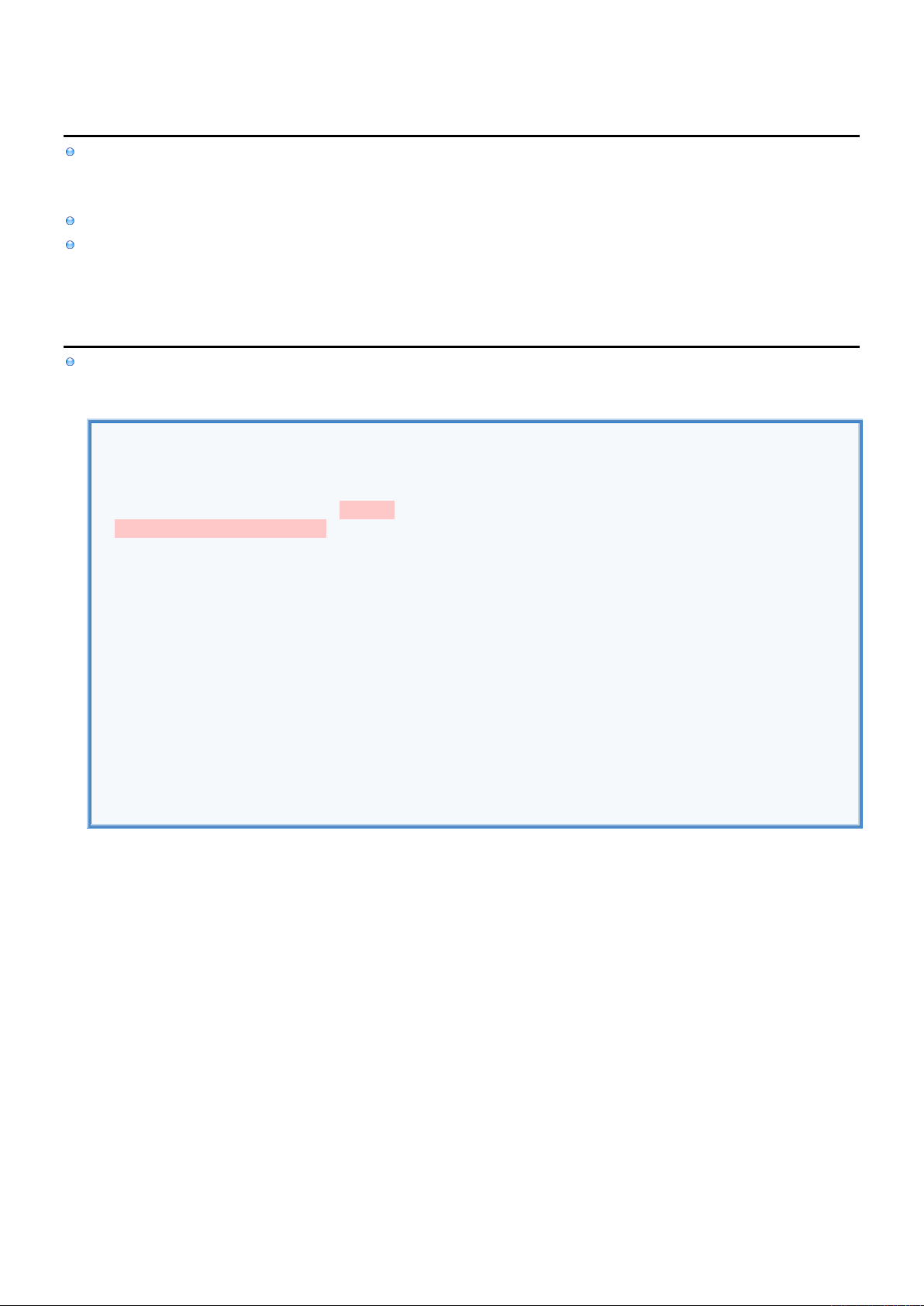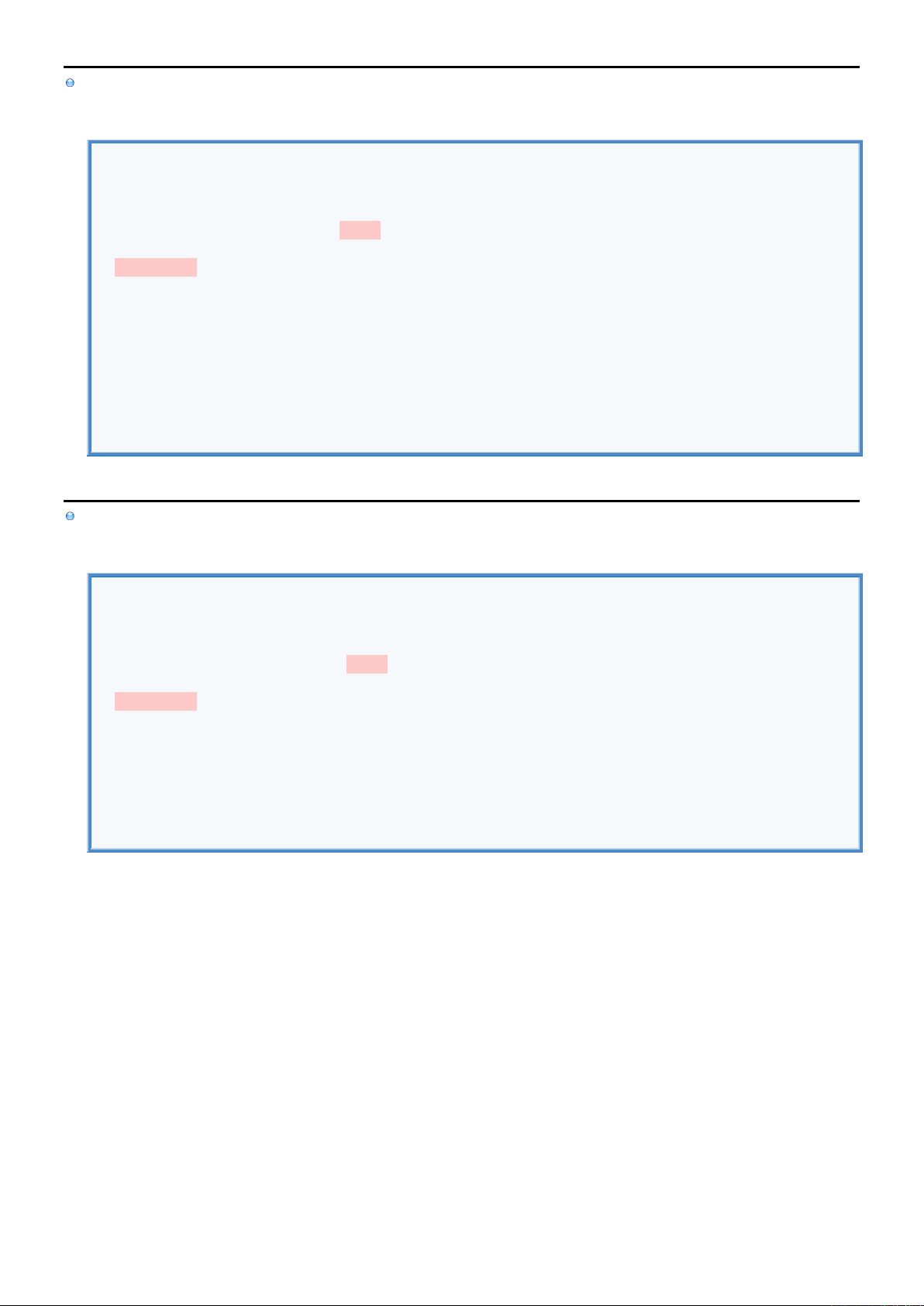2
2
.
.
1
1
.
.
4
4
C
C
u
u
s
s
t
t
o
o
m
m
O
O
p
p
e
e
r
r
a
a
t
t
o
o
r
r
s
s
I
I
n
n
f
f
o
o
Custom Operators allow you to specify custom operations that should be performed on
two Variables (Infix Custom Operators: john + bob)
single Variable (Prefix & Postfix Custom Operators: +++age, age+++)
First we need to declare the operator as a global variable before we can write its implementation.
Highlighted parts are added so that operator would also return the value (allowing us to store it into another variable).
Without them Prefix & Postfix Operators would only change the value of the Variable on which they were applied.
And in the case of Infix Operators there would be also no return Value.
I
I
n
n
f
f
i
i
x
x
O
O
p
p
e
e
r
r
a
a
t
t
o
o
r
r
In this example we define "+" operator that adds score Properties of two Players structs.
Infix Operator
//DECLARE OPERATOR.
infix operator +
//IMPLEMENT OPERATOR.
func + (left:Player, right:Player) -> (Int) {
return left.score + right.score
}
//DECLARE STRUCT.
struct Player {
var name : String
var score : Int
}
//USE OPERATOR.
var john = Player(name: "John", score: 5)
var bob = Player(name: "Bob" , score: 2)
var totalScore = john + bob //7 Custom Operation. Add scores.
//DISPLAY RESULT.
print(totalScore)P
P
r
r
e
e
f
f
i
i
x
x
O
O
p
p
e
e
r
r
a
a
t
t
o
o
r
r
In this example we define "+++" Prefix Operator that works on Integer to double its value.
Prefix Operator
//DECLARE OPERATOR.
prefix operator +++
//IMPLEMENT OPERATOR.
prefix func +++ (value: inout Int) -> Int {
value += value
return value
}
//USE OPERATOR.
var age = 50
var result : Int = +++age //Double the value of age. Return result.
//DISPLAY VARIABLES.
print(age)
print(result)
P
P
o
o
s
s
t
t
f
f
i
i
x
x
O
O
p
p
e
e
r
r
a
a
t
t
o
o
r
r
In this example we define "+++" Postfix Operator that works on Integer to double its value.
Postfix Operator
//DECLARE OPERATOR.
postfix operator +++
//IMPLEMENT OPERATOR.
postfix func +++ (value: inout Int) -> Int {
value += value
return value
}
//USE OPERATOR.
var age = 50
var age2:Int = age+++ //Double the value of age. Return doubled value.
print(age )
print(age2)